# scipy.spatial.distance.sokalmichener¶

scipy.spatial.distance.sokalmichener(u, v)

Computes the Sokal-Michener dissimilarity between two boolean vectors u and v, which is defined as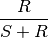where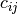is the number of occurrences of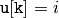and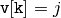for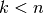,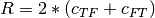and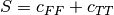.

Parameters : u : ndarray An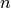-dimensional vector. v : ndarray An-dimensional vector. d : double The Sokal-Michener dissimilarity between vectors u and v.

#### Previous topic

scipy.spatial.distance.seuclidean

#### Next topic

scipy.spatial.distance.sokalsneath